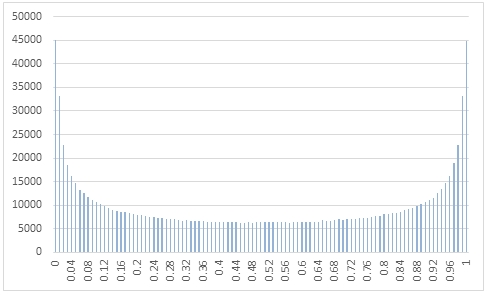# SQL Server random beta distribution

RANDBETA

Updated: 31 March 2014

Use the table-valued function RANDBETA to generate q sequence of random numbers from the beta distribution with two positive shape parameters @a and @b.
Syntax
SELECT * FROM [wctMath].[wct].[RANDBETA](
<@Rows, int,>
,<@a, float,>
,<@b, float,>)
Arguments
@Rows
the number of rows to generate. @Rows must be of the type int or of a type that implicitly converts to int.
@a
the first shape parameter. @a must be of the type float or of a type that implicitly converts to float.
@b
the second shape parameter. @b must be of the type float or of a type that implicitly converts to float.
Return Types
RETURNS TABLE (
[Seq] [int] NULL,
[X] [float] NULL
)
Remarks
·         @a must be greater than zero.
·         @b must be greater than zero.
·         If @a is NULL then @a is set to 1.
·         If @b is NULL then @b is set to 1.
·         If @Rows is less than 1 then no rows are returned.
Examples
In this example we create a sequence 1,000,000 random numbers rounded to two decimal places from a beta distribution with @a = 0.5 and @b = 0.5, COUNT the result, paste them into Excel and graph them.
SELECT
X,
COUNT(*) as [COUNT]
FROM (
SELECT
ROUND(x,2) as X
FROM wct.RANDBETA(
1000000, --@Rows
0.5,     --@a
0.5      --@b
)
)n
GROUP BY
X
ORDER BY
1

This produces the following result.In this example we generate 1,000,000 random numbers from a beta distribution with
@a of 2 and @b of 5. We calculate the mean, standard deviation, skewness, and excess kurtosis from the resultant table and compare those values to the expected values for the distribution.
DECLARE @size as int = 1000000
DECLARE @a as float = 2
DECLARE @b as float = 5
DECLARE @mean as float = @a/(@a+@b)
DECLARE @var as float =(@a*@b)/(POWER(@a+@b,2)*(@a+@b+1))
DECLARE @stdev as float = SQRT(@var)
DECLARE @skew as float =(2*(@b-@a)*SQRT(@a+@b+1))/((@a+@b+2)*SQRT(@a*@b))
DECLARE @kurt as float =(6*(POWER(@a-@b,2)*(@a+@b+1)-@a*@b*(@a+@b+2)))/(@a*@b*(@a+@b+2)*(@a+@b+3))

SELECT
stat,
[RANDBETA],
[EXPECTED]
FROM (
SELECT
x.*
FROM (
SELECT
AVG(x) as mean_BETA,
STDEVP(x) as stdev_BETA,
wct.SKEWNESS_P(x) as skew_BETA,
wct.KURTOSIS_P(x) as kurt_BETA
FROM
wct.RANDBETA(@size,@a,@b)
)n
CROSS APPLY(
VALUES
('RANDBETA','avg', mean_BETA),
('RANDBETA','stdev', stdev_BETA),
('RANDBETA','skew', skew_BETA),
('RANDBETA','kurt', kurt_BETA),
('EXPECTED','avg',@mean),
('EXPECTED','stdev',@stdev),
('EXPECTED','skew',@skew),
('EXPECTED','kurt',@kurt)
)x(fn_name,stat,val_stat)
)d
PIVOT(sum(val_stat) FOR fn_name in([RANDBETA],[EXPECTED])) P

This produces the following result (your result will be different).

 stat RANDBETA EXPECTED avg 0.285978037 0.285714286 kurt -0.126404207 -0.12 skew 0.593719846 0.596284794 stdev 0.159859385 0.159719141

### SupportCopyright 2008-2023 Westclintech LLC         Privacy Policy        Terms of Service